# Push Relabel Algorithm | Set 1 (Introduction and Illustration)

• Difficulty Level : Hard
• Last Updated : 08 Apr, 2022

Given a graph which represents a flow network where every edge has a capacity. Also given two vertices source ‘s’ and sink ‘t’ in the graph, find the maximum possible flow from s to t with following constraints: a) Flow on an edge doesn’t exceed the given capacity of the edge. b) Incoming flow is equal to outgoing flow for every vertex except s and t. For example, consider the following graph from CLRS book.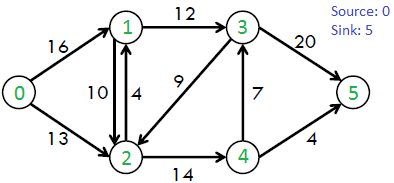The maximum possible flow in the above graph is 23.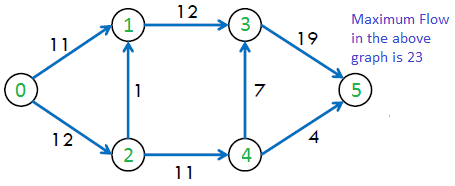We have discussed Ford Fulkerson Algorithm that uses augmenting path for computing maximum flow.

Push-Relabel Algorithm

Push-Relabel approach is the more efficient than Ford-Fulkerson algorithm. In this post, Goldberg’s “generic” maximum-flow algorithm is discussed that runs in O(V2E) time. This time complexity is better than O(E2V) which is time complexity of Edmond-Karp algorithm (a BFS based implementation of Ford-Fulkerson). There exist a push-relabel approach based algorithm that works in O(V3) which is even better than the one discussed here. Similarities with Ford Fulkerson

• Like Ford-Fulkerson, Push-Relabel also works on Residual Graph (Residual Graph of a flow network is a graph which indicates additional possible flow. If there is a path from source to sink in residual graph, then it is possible to add flow).

Differences with Ford Fulkerson

• Push-relabel algorithm works in a more localized. Rather than examining the entire residual network to find an augmenting path, push-relabel algorithms work on one vertex at a time (Source : CLRS Book).
• In Ford-Fulkerson, net difference between total outflow and total inflow for every vertex (Except source and sink) is maintained 0. Push-Relabel algorithm allows inflow to exceed the outflow before reaching the final flow. In final flow, the net difference is 0 for all except source and sink.
• Time complexity wise more efficient.

The intuition behind the push-relabel algorithm (considering a fluid flow problem) is that we consider edges as water pipes and nodes are joints. The source is considered to be at the highest level and it sends water to all adjacent nodes. Once a node has excess water, it pushes water to a smaller height node. If water gets locally trapped at a vertex, the vertex is Relabeled which means its height is increased. Following are some useful facts to consider before we proceed to algorithm.

• Each vertex has associated to it a height variable and a Excess Flow. Height is used to determine whether a vertex can push flow to an adjacent or not (A vertex can push flow only to a smaller height vertex). Excess flow is the difference of total flow coming into the vertex minus the total flow going out of the vertex.
```     Excess Flow of u = Total Inflow to u -
Total Outflow from u```
• Like Ford Fulkerson. each edge has associated to it a flow (which indicates current flow) and a capacity

Following are abstract steps of complete algorithm.

```Push-Relabel Algorithm
1) Initialize PreFlow : Initialize Flows
and Heights

2) While it is possible to perform a Push() or
Relabel() on a vertex
// Or while there is a vertex that has excess flow
Do Push() or Relabel()

// At this point all vertices have Excess Flow as 0 (Except source
// and sink)
3) Return flow.```

There are three main operations in Push-Relabel Algorithm

1. Initialize PreFlow() It initializes heights and flows of all vertices.
```Preflow()
1) Initialize height and flow of every vertex as 0.
2) Initialize height of source vertex equal to total
number of vertices in graph.
3) Initialize flow of every edge as 0.
4) For all vertices adjacent to source s, flow and
excess flow is equal to capacity initially.```
1. Push() is used to make the flow from a node which has excess flow. If a vertex has excess flow and there is an adjacent with smaller height (in residual graph), we push the flow from the vertex to the adjacent with lower height. The amount of pushed flow through the pipe (edge) is equal to the minimum of excess flow and capacity of edge.
2. Relabel() operation is used when a vertex has excess flow and none of its adjacent is at lower height. We basically increase height of the vertex so that we can perform push(). To increase height, we pick the minimum height adjacent (in residual graph, i.e., an adjacent to whom we can add flow) and add 1 to it.

Note that above operations are performed on residual graph (like Ford-Fulkerson).  Illustration:Before we proceed to below example, we need to make sure that we understand residual graph (See this for more details of residual graph). Residual graph is different from graphs shown. Whenever we push or add a flow from a vertex u to v, we do following updates in residual graph : 1) We subtract the flow from capacity of edge from u to v. If capacity of an edge becomes 0, then the edge no longer exists in residual graph. 2) We add flow to the capacity of edge from v to u.

```For example, consider two vertices u an v.

In original graph
3/10
u ---------> v
3 is current flow from u to v and
10 is capacity of edge from u to v.

In residual Graph, there are two edges corresponding
to one edge shown above.
7
u ---------> v

3
u <--------- v ```
1. Initial given flow graph.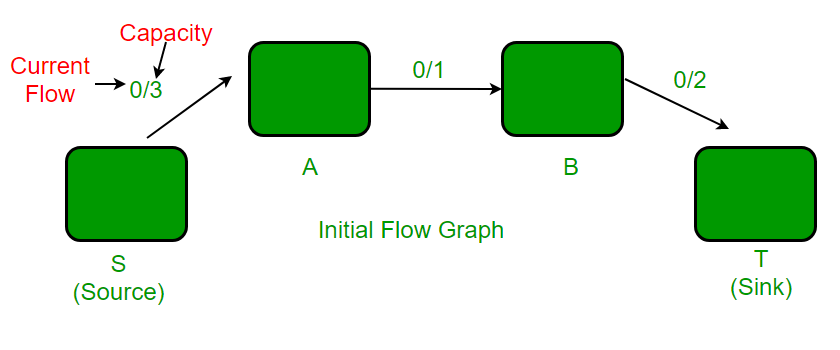2. After PreFlow operation. In residual graph, there is an edge from A to S with capacity 3 and no edge from S to A.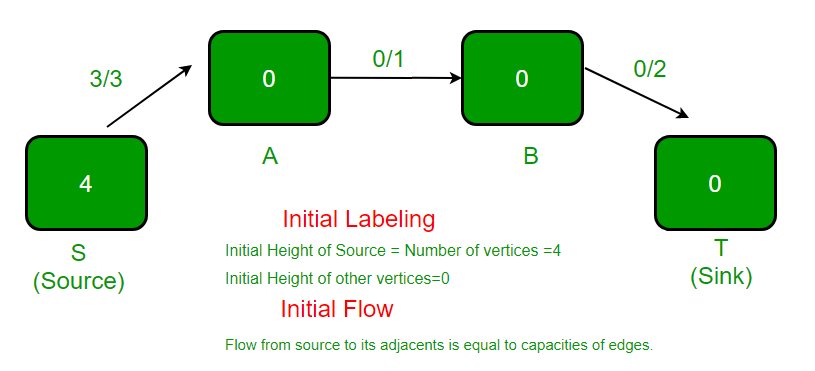3. The highlighted vertex is relabeled (height becomes 1) as it has excess flow and there is no adjacent with smaller height. The new height is equal to minimum of heights of adjacent plus 1.  In residual graph, there are two adjacent of vertex A, one is S and other is B.  Height of S is 4 and height of B is 0.  Minimum of these two heights is 0. We take the minimum and add 1 to it.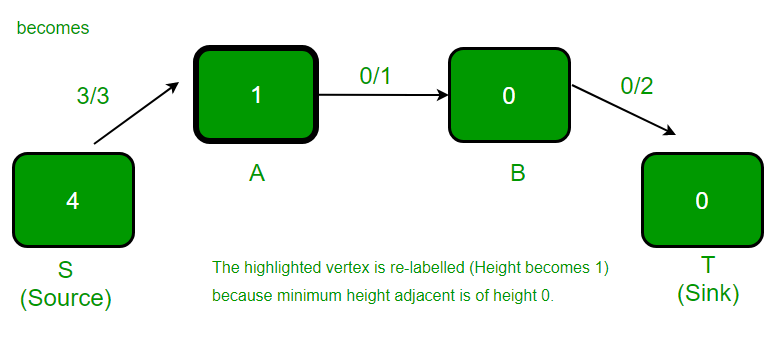4. The highlighted vertex has excess flow and there is an adjacent with lower height, so push() happens. Excess flow of vertex A is 2 and capacity of edge (A, B) is 1. Therefore, the amount of pushed flow is 1 (minimum of two values).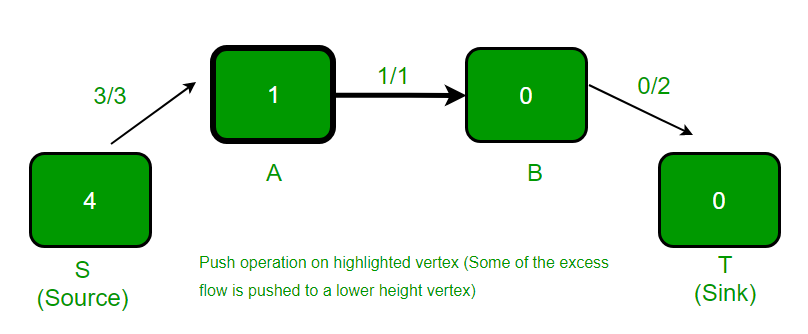5. The highlighted vertex is relabeled (height becomes 1) as it has excess flow and there is no adjacent with smaller height.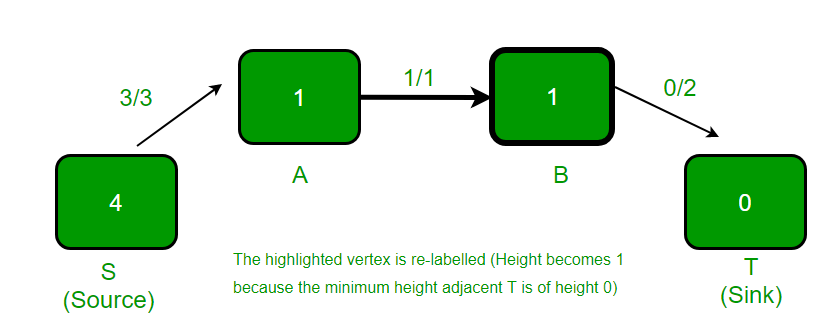6. The highlighted vertex has excess flow and there is an adjacent with lower height, so flow() is pushed from B to T.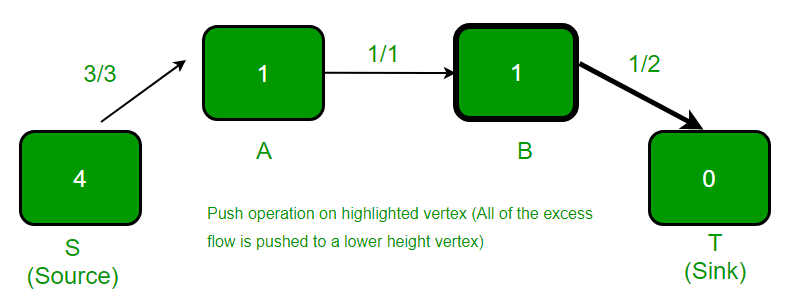7. The highlighted vertex is relabeled (height becomes 5) as it has excess flow and there is no adjacent with smaller height.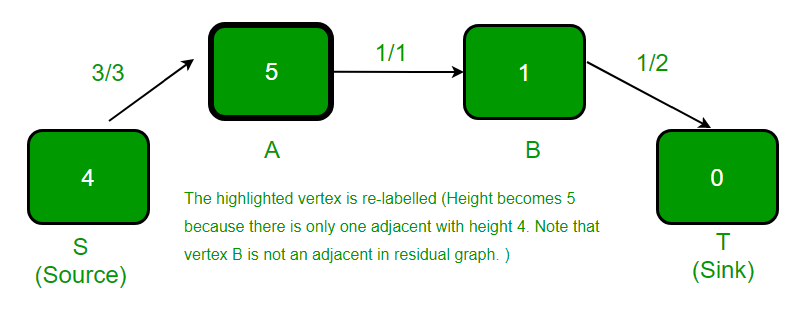8. The highlighted vertex has excess flow and there is an adjacent with lower height, so push() happens.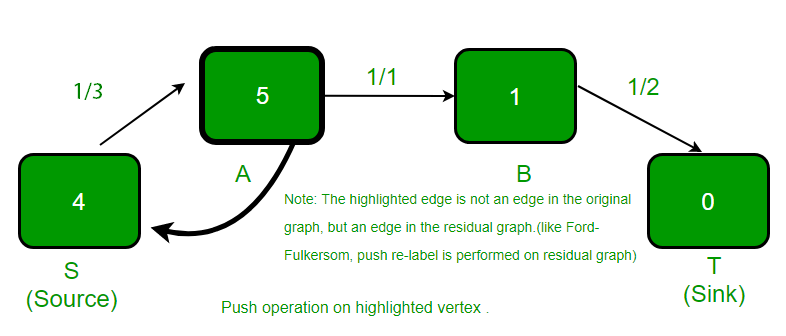9. The highlighted vertex is relabeled (height is increased by 1) as it has excess flow and there is no adjacent with smaller height.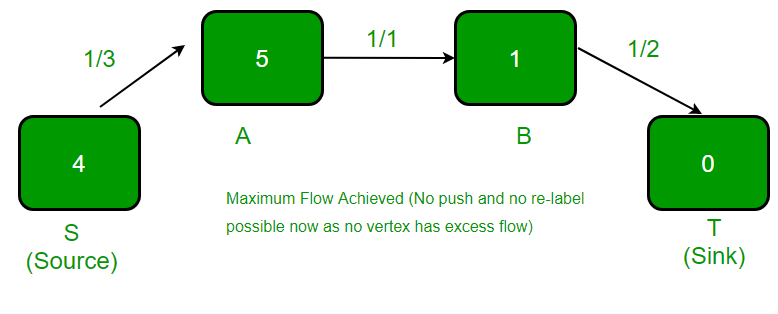My Personal Notes arrow_drop_up